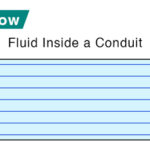Home / Physics / Viscosity

# Viscosity

Viscosity is a physical quantity that describes a fluid’s resistance to flow. It is a property that resists the relative displacement of the different layers of the fluid. It can be considered as the fluid friction occurring inside the fluid due to the internal friction between the molecules. Viscosity is a primary factor in determining the forces to overcome when fluids are used in lubrication.

## High Viscosity vs. Low Viscosity

Viscosity can be grouped into two categories based on fluid flow resistance – high and low. A high-viscosity fluid will be more viscous than a low-viscosity fluid. Fluids with low viscosity have a low resistance, shear quickly, and the molecules flow rapidly. On the other hand, high-viscosity fluids move languidly and resist deformation.

Examples

High-viscosity fluids include honey, pitch, molten glass, and peanut butter; low-viscosity fluids include air and water.

## Dynamic and Kinematic Viscosity

There are two types of viscosity – dynamic and kinematic. Dynamic viscosity or absolute viscosity is the fluid’s resistance to motion when an external force is applied to shear the fluid. Kinematic viscosity is the resistive flow of fluid under the action of gravity.

Two fluids having the same dynamic viscosity can have different kinematic viscosities. The reason is that kinematic viscosity depends on the density of the fluid. On the other hand, density is not a factor with dynamic viscosity.

## Formula

Dynamic Viscosity

Since viscosity measures the resistance of a fluid deformed by shear, it is expressed as the ratio of the shear stress to the velocity gradient.

$\eta = \frac{F}{A\big (\frac{\partial u}{\partial y}\big)}$

Where

η is the dynamic viscosity

F is the applied force

A is the area over which the force is applied

The above equation was derived by British mathematician Isaac Newton and is called Newton’s law of viscosity. It is applicable for straight, parallel, and uniform flow. The term u/v is the shear rate of deformation, and du/dv is the shear velocity.

Kinematic Viscosity

The ratio of the dynamic viscosity and fluid density gives the kinematic viscosity.

$\mu = \frac{\eta }{\rho }$

Where

ν is the kinematic viscosity

η is the dynamic viscosity

ρ is the density

### Symbols and Units

Dynamic Viscosity

Greek symbol mu (μ) or eta (η) represents dynamic viscosity. Its SI unit is Pascal-second or Paˑs, equivalent to Nˑs/m2 or Paˑs. The cgs unit is poise or P. ASTM standard uses centiPoise or cP.

1 P = 0.1 Paˑs and 1 cP = 0.001 Paˑs

Kinematic Viscosity

Greek symbol nu (ν) represents kinematic viscosity. The SI unit of kinematic viscosity is m2/s. The cgs unit is Stokes or St. It is sometimes expressed in centiStokes.

1 St = 10-4 m2/s and 1 cSt = 10-6 m2/s

## Viscosity Chart

Viscosity is measured using a viscometer. The following table gives the viscosity values of some common substances.

Chart Courtesy: Innovative Calibration Solutions

## Factors Affecting Viscosity

The viscosity of liquids decreases quickly with an increase in temperature. On the other hand, the viscosity of gases increases with an increase in temperature. Thus, liquids flow more smoothly upon heating, and gasses flow more sluggishly.

The viscosity of gases is approximately proportional to the square root of temperature. The reason why gases increase their viscosity is because of an increased frequency of collisions. The more the molecules collide, the more disorganized they become. As a result, they move sluggishly.

Intermolecular forces in a fluid are a factor that affects viscosity. The higher the intermolecular forces, the higher the viscosity. Due to strong intermolecular forces, the fluid molecules are strongly bonded to each other. As a result, they are prevented from moving.

## Newtonian and Non-Newtonian Fluid

It has been found that the viscosity is independent of the strain rates for a wide range of fluids. It means the viscosity remains constant no matter how much force is applied. Such a type of fluid is known as Newtonian fluid. The relationship between the viscosity and shear stress in a Newtonian fluid is linear. Examples are water, mineral oil, alcohol, and gasoline.

On the other hand, if the viscosity does not remain constant and depends on the force applied, the fluid is called non-Newtonian fluid. The viscosity changes as shear stress is applied. Examples include slime, toothpaste, cosmetics, and paints.

Article was last reviewed on Thursday, April 6, 2023

### Related articlesLaminar FlowRadius of GyrationNewton’s Law of CoolingVelocity